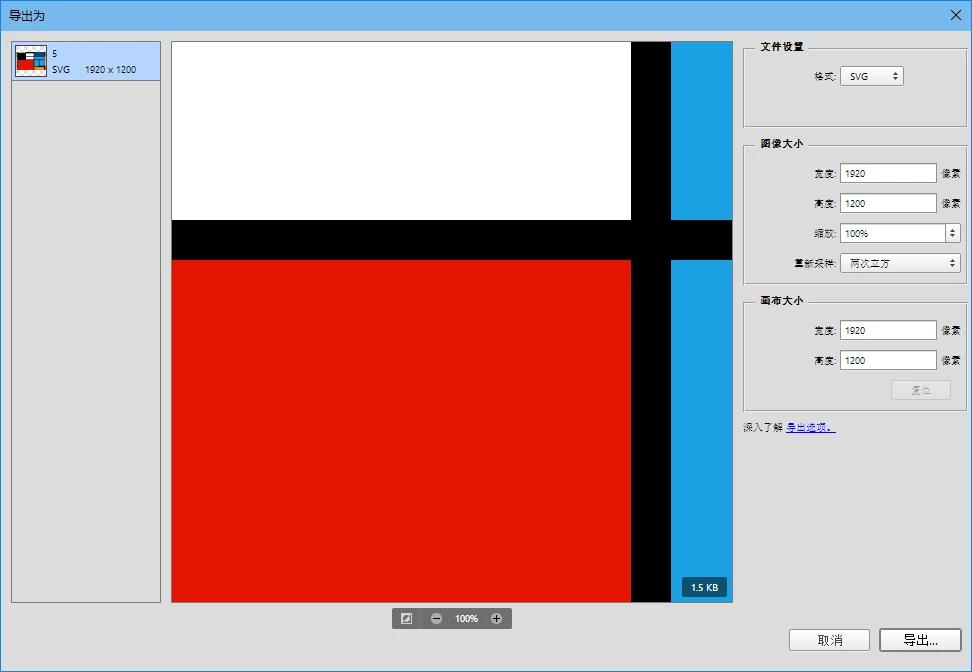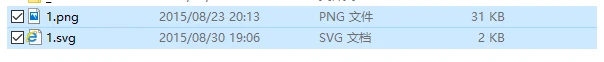# SVG图形应用一例：焕蓝主页采用SVG

• SVG 指可伸缩矢量图形 (Scalable Vector Graphics)
• SVG 用来定义用于网络的基于矢量的图形
• SVG 使用 XML 格式定义图形
• SVG 图像在放大或改变尺寸的情况下其图形质量不会有所损失
• SVG 是万维网联盟的标准
• SVG 与诸如 DOM 和 XSL 之类的 W3C 标准是一个整体

• SVG 可被非常多的工具读取和修改（比如记事本）
• SVG 与 JPEG 和 GIF 图像比起来，尺寸更小，且可压缩性更强。
• SVG 是可伸缩的
• SVG 图像可在任何的分辨率下被高质量地打印
• SVG 可在图像质量不下降的情况下被放大
• SVG 图像中的文本是可选的，同时也是可搜索的（很适合制作地图）
• SVG 可以与 Java 技术一起运行
• SVG 是开放的标准
• SVG 文件是纯粹的 XML

1. 首先，也是最重要的，如果要导出SVG，尽量在作图时使用路径作图，不要栅格化，否则导出的SVG仍然很大，因为如果用路径作图，PS将会将其视为矢量图，可以使用SVG定义的多种标签进行绘图，导出的SVG很小；如果栅格化，PS将会将其视为位图，导出内容包含各个栅格化图层的Base64代码，其大小可想而知。
2. 做好图后，依次点击“ 文件 > 导出 > 导出为… ”,弹出“导出为”窗口，如图：3. 选择“文件设置”下的“格式”为SVG，设置其他信息。注意：由于SVG为矢量图，更改尺寸并不能显著改变文件大小，反而可能会出现一些意外的结果（如：周围有透明空白），所以不建议更改尺寸。
4. 单击“导出…”按钮，选择所需路径，编辑好文件名，保存。``````<svg xmlns="http://www.w3.org/2000/svg" xmlns:xlink="http://www.w3.org/1999/xlink" preserveAspectRatio="xMidYMid" width="1920" height="1200" viewBox="0 0 1920 1200">
<defs>
<style>
.cls-1 {
fill: #000;
}

.cls-2 {
fill: #e51400;
}

.cls-3 {
fill: #de9317;
}

.cls-4 {
fill: #034888;
}

.cls-5 {
fill: #1ba1e2;
}

.cls-6 {
fill: #fff;
}
</style>
</defs>
<rect width="1920" height="1200" class="cls-1"/>
<rect x="1867" width="53" height="267" class="cls-2"/>
<rect x="1179" y="997" width="648" height="203" class="cls-3"/>
<rect x="1179" width="648" height="267" class="cls-4"/>
<rect x="1179" y="307" width="648" height="191" class="cls-5"/>
<rect x="1179" y="538" width="304" height="419" class="cls-5"/>
<rect x="1523" y="538" width="304" height="379" class="cls-5"/>
<rect width="42" height="267" class="cls-3"/>
<rect x="82" width="504" height="48" class="cls-6"/>
<rect y="307" width="42" height="650" class="cls-6"/>
<rect y="997" width="42" height="203" class="cls-6"/>
<rect x="626" y="87" width="513" height="180" class="cls-6"/>
<rect x="626" y="307" width="513" height="191" class="cls-6"/>
<rect x="1867" y="307" width="53" height="610" class="cls-6"/>
<rect x="1867" y="997" width="53" height="203" class="cls-2"/>
<rect x="82" y="538" width="1057" height="662" class="cls-2"/>
</svg>``````

目录